# Prediction Tutorial¶

Simulates “true data” corrected by acceptance, according to
the fitted values for the amplitude
:

import PyPWA as pwa
import numpy as npy
import pandas
import matplotlib.pyplot as plt
import warnings
warnings.filterwarnings('ignore')


## Define waves and amplitude (function) to simulate¶

:

initial=[]
for param_type in ["r", "i"]:
initial.append(f"{param_type}.1.0.0")
initial.append(f"{param_type}.1.1.0")
initial.append(f"{param_type}.1.1.1")
initial.append(f"{param_type}.1.2.0")
initial.append(f"{param_type}.1.2.1")
initial.append(f"{param_type}.1.2.2")
#import AmplitudeOLDfit
#amp = AmplitudeOLDfit.FitAmplitude(initial)
#
import AmplitudeJPACsim
amp = AmplitudeJPACsim.NewAmplitude()
#
#import AmplitudeJPACfit
#amp = AmplitudeJPACfit.FitAmplitude(initial)


## Read input (flat) simulated (generated) data¶

:

data = pwa.read("etapiHEL_flat.txt")


Read flat data in gamp format (full information)

:

datag = pwa.read("../TUTORIAL_FILES/raw_events.gamp")


Define number of bins (MUST be the same as the fitted parameters)

:

nbins=20
bins = pwa.bin_by_range(data, "mass", nbins, .6, 2.0)


Calculate the mass value and number of events in each bin

:

bmass=[]
mcounts=[]
for index, bin in enumerate(bins):
if len(bin)==0:
bmass.append(0.)
mcounts.append(0.)
else:
bmass.append(npy.average(bin["mass"]))
mcounts.append(len(bin))


:

par = pwa.read("final_values_JPAC.csv")


Prepare for a binned simulation > Find intensities in each bin and max intensity for each bin.

:

int_values = []
max_values = []
params={}
for index, bin in enumerate(bins):
for param_type in ["r", "i"]:
params.update({f"{param_type}.1.0.0":par[f"{param_type}.1.0.0"][index]})
params.update({f"{param_type}.1.1.0":par[f"{param_type}.1.1.0"][index]})
params.update({f"{param_type}.1.1.1":par[f"{param_type}.1.1.1"][index]})
params.update({f"{param_type}.1.2.0":par[f"{param_type}.1.2.0"][index]})
params.update({f"{param_type}.1.2.1":par[f"{param_type}.1.2.1"][index]})
params.update({f"{param_type}.1.2.2":par[f"{param_type}.1.2.2"][index]})

[int,intmax] = pwa.simulate.process_user_function(amp,bin,params,16)
int_values.append(int)
max_values.append(intmax)


:

rejected_bins = []
for int_value, bin in zip(int_values, bins):
rejection = pwa.simulate.make_rejection_list(int_value, max_values)
rejected_bins.append(bin[rejection])


Check how many events simulated by bin

:

for index, simulated_bin in enumerate(rejected_bins):
print(
f"Bin {index+1}'s length is {len(simulated_bin)}, "
f"{(len(simulated_bin) / len(bin)) * 100:.2f}% events were kept"
)

Bin 1's length is 4850, 1.79% events were kept
Bin 2's length is 6545, 2.42% events were kept
Bin 3's length is 10388, 3.84% events were kept
Bin 4's length is 25492, 9.42% events were kept
Bin 5's length is 65913, 24.34% events were kept
Bin 6's length is 27495, 10.15% events were kept
Bin 7's length is 15419, 5.69% events were kept
Bin 8's length is 17872, 6.60% events were kept
Bin 9's length is 31916, 11.79% events were kept
Bin 10's length is 45793, 16.91% events were kept
Bin 11's length is 20121, 7.43% events were kept
Bin 12's length is 10427, 3.85% events were kept
Bin 13's length is 10301, 3.80% events were kept
Bin 14's length is 12541, 4.63% events were kept
Bin 15's length is 15215, 5.62% events were kept
Bin 16's length is 18517, 6.84% events were kept
Bin 17's length is 17288, 6.39% events were kept
Bin 18's length is 12478, 4.61% events were kept
Bin 19's length is 8226, 3.04% events were kept
Bin 20's length is 5718, 2.11% events were kept


Stak data for all bins in one file (new_data)

:

for index, the_bin in zip(range(len(rejected_bins)), rejected_bins):
if index ==0:
new_data=pandas.DataFrame(the_bin)

new_data =  new_data.append(the_bin,ignore_index=True)

#print(new_data)

:

new_data

:

EventN theta phi alpha pol tM mass
0 432.0 1.075030 3.11080 -2.279760 0.4 -0.036087 0.730692
1 3277.0 1.106290 1.62386 2.912540 0.4 -0.021695 0.706537
2 5034.0 2.092900 1.77176 -1.243810 0.4 -0.065768 0.730773
3 5547.0 0.201674 -1.60246 -1.418660 0.4 -0.028844 0.701134
4 6527.0 1.325940 3.00931 -1.637510 0.4 -0.023814 0.722924
... ... ... ... ... ... ... ...
387360 9849089.0 1.940630 -1.85137 1.307640 0.4 -0.521166 1.968390
387361 9851359.0 1.055120 -2.61521 -2.743190 0.4 -0.403025 1.960020
387362 9853817.0 0.189324 -1.00286 -1.850720 0.4 -0.370487 1.944500
387363 9854636.0 1.043920 2.97397 -0.604751 0.4 -0.325758 1.971750
387364 9854972.0 0.607401 -1.62378 -1.638410 0.4 -0.282602 1.978580

387365 rows × 7 columns

## Calculate the number of expected events versus mass bins¶

:

total_nExp = npy.empty(len(rejected_bins))
for index, the_bin in zip(range(len(rejected_bins)), rejected_bins):

for param_type in ["r", "i"]:
params.update({f"{param_type}.1.0.0":par[f"{param_type}.1.0.0"][index]})
params.update({f"{param_type}.1.1.0":par[f"{param_type}.1.1.0"][index]})
params.update({f"{param_type}.1.1.1":par[f"{param_type}.1.1.1"][index]})
params.update({f"{param_type}.1.2.0":par[f"{param_type}.1.2.0"][index]})
params.update({f"{param_type}.1.2.1":par[f"{param_type}.1.2.1"][index]})
params.update({f"{param_type}.1.2.2":par[f"{param_type}.1.2.2"][index]})
amp.setup(the_bin)
total_nExp[index] = npy.average(amp.calculate(params))


Plot number of true (100% acc) events versus mass

:

mni = npy.empty(len(total_nExp), dtype=[("mass", float), ("int", float)])
mni["mass"] = bmass
mni["int"] = total_nExp
mni = pandas.DataFrame(mni)
counts, bin_edges = npy.histogram(mni["mass"], nbins, weights=mni["int"])

centers = (bin_edges[:-1] + bin_edges[1:]) / 2

# Add yerr to argment list when we have errors
yerr = npy.empty(nbins)
yerr = npy.sqrt(counts)
yerr=0.
plt.errorbar(centers,counts, yerr, fmt="s",linestyle="dashed",markersize='10',label="nExp")

#plt.xlim(.6, 3.2)
#plt.ylim(0.,65000)
plt.legend(loc='upper right')
plt.show()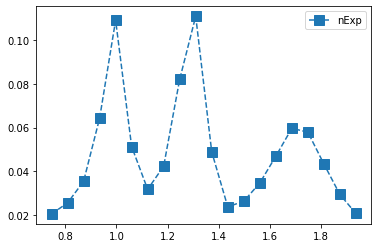## Calculate Phase difference between two waves¶

:

V1=npy.ndarray(len(par))
V2=npy.ndarray(len(par))
V1 = par["r.1.1.1"]+par["i.1.1.1"]*(1j)
V2 = par["r.1.2.1"]+par["i.1.2.1"]*(1j)
if V1.all() != 0 and V2.all() != 0:
phasediff = npy.arctan(npy.imag(V1*npy.conj(V2))/npy.real(V1*npy.conj(V2)))


Plot PhaseMotion

:

mnip = npy.empty(len(bins), dtype=[("mass", float), ("phase", float)])
mnip["mass"] = bmass
mnip["phase"] = phasediff
mnip = pandas.DataFrame(mnip)
counts, bin_edges = npy.histogram(mnip["mass"], 25, weights=mnip["phase"])
centers = (bin_edges[:-1] + bin_edges[1:]) / 2

# Add yerr to argment list when we have errors
yerr = npy.empty(100)
yerr = npy.sqrt(npy.abs(counts))
plt.errorbar(centers,counts, yerr, fmt="o")
plt.xlim(0.6, 3.2)

:

(0.6, 3.2)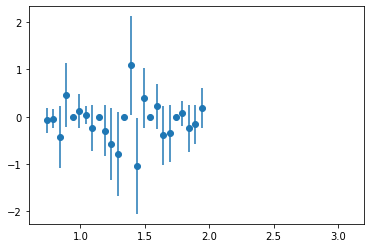Plot phi_HEL vs cos(theta_HEL) of predicted data (with 4 different contracts(gamma))

:

import matplotlib.colors as mcolors
from numpy.random import multivariate_normal

gammas = [0.8, 0.5, 0.3]

fig, axes = plt.subplots(nrows=2, ncols=2)

axes[0, 0].set_title('Linear normalization')
axes[0, 0].hist2d(new_data["phi"], npy.cos(new_data["theta"]), bins=100)
#axes[0, 0].hist2d(cut_list["phi"], npy.cos(cut_list["theta"]), bins=100)

for ax, gamma in zip(axes.flat[1:], gammas):
ax.set_title(r'Power law $(\gamma=%1.1f)$' % gamma)
ax.hist2d(new_data["phi"], npy.cos(new_data["theta"]),
bins=100, norm=mcolors.PowerNorm(gamma))

fig.tight_layout()

plt.show()Plot cos(theta_HEL) vs mass for simulated data (with 4 different contrasts)

:

gammas = [0.8, 0.5, 0.3]

fig, axes = plt.subplots(nrows=2, ncols=2)

axes[0, 0].set_title('Linear normalization')
axes[0, 0].hist2d(new_data["mass"], npy.cos(new_data["theta"]),bins=100)

for ax, gamma in zip(axes.flat[1:], gammas):
ax.set_title(r'Power law $(\gamma=%1.1f)$' % gamma)
ax.hist2d(new_data["mass"], npy.cos(new_data["theta"]),
bins=100, norm=mcolors.PowerNorm(gamma))

fig.tight_layout()
plt.xlim(.6, 2.)
plt.show()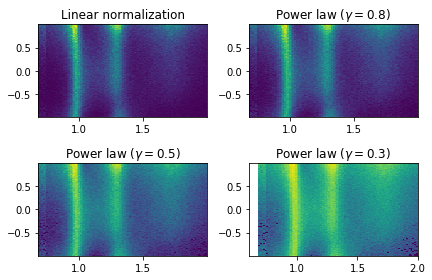Plot phi_HEL vs mass for simulated data (with 4 different contrasts)

:

gammas = [0.8, 0.5, 0.3]

fig, axes = plt.subplots(nrows=2, ncols=2)

axes[0, 0].set_title('Linear normalization')
axes[0, 0].hist2d(new_data["mass"], new_data["phi"],bins=100)

for ax, gamma in zip(axes.flat[1:], gammas):
ax.set_title(r'Power law $(\gamma=%1.1f)$' % gamma)
ax.hist2d(new_data["mass"], new_data["phi"],
bins=100, norm=mcolors.PowerNorm(gamma))

fig.tight_layout()
plt.xlim(.6, 2.)
plt.show()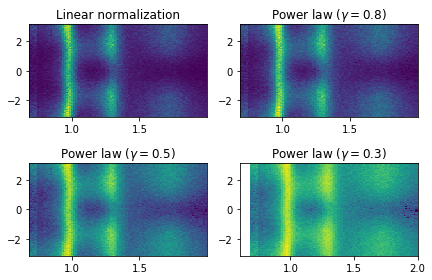[ ]:



:

plt.hist(new_data["alpha"],50)
plt.show()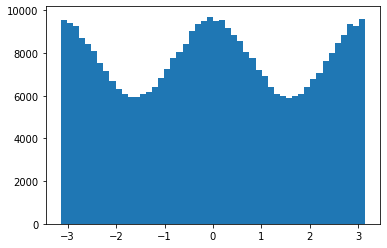Plot mass versus alpha/Phi

:

gammas = [0.8, 0.5, 0.3]

fig, axes = plt.subplots(nrows=2, ncols=2)

axes[0, 0].set_title('Linear normalization')
axes[0, 0].hist2d(new_data["mass"], new_data["alpha"],bins=100)

for ax, gamma in zip(axes.flat[1:], gammas):
ax.set_title(r'Power law $(\gamma=%1.1f)$' % gamma)
ax.hist2d(new_data["mass"], new_data["alpha"],
bins=100, norm=mcolors.PowerNorm(gamma))

fig.tight_layout()
plt.xlim(.6, 2.)
plt.show()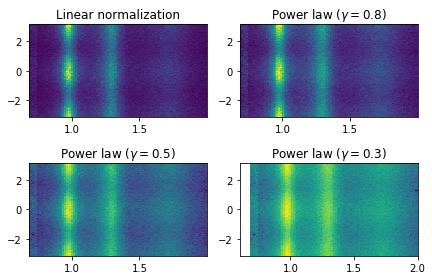Plot mass versus alpha/Phi

:

gammas = [0.8, 0.5, 0.3]

fig, axes = plt.subplots(nrows=2, ncols=2)

axes[0, 0].set_title('Linear normalization')
axes[0, 0].hist2d(new_data["phi"],new_data["alpha"],bins=100)

for ax, gamma in zip(axes.flat[1:], gammas):
ax.set_title(r'Power law $(\gamma=%1.1f)$' % gamma)
ax.hist2d(new_data["phi"], new_data["alpha"],
bins=100, norm=mcolors.PowerNorm(gamma))

fig.tight_layout()

plt.show()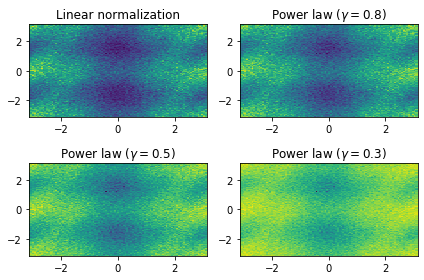## Write predicted (true) data to disk¶

:

new_data.to_csv("predictedjpac.csv", index=False)


Write gamp format predicted to data (true)

:

x = (data["EventN"]).astype(int)
y = (new_data["EventN"]).astype(int)

predm = npy.isin(x,y)
pdatag = datag[predm]
pwa.write("raw_predicted.gamp",pdatag)


Write predicted accepted data > events.pf is a mask of accepted data that has been produced by Geant

:

acc = pwa.read("events.pf")
accn = acc.to_numpy()